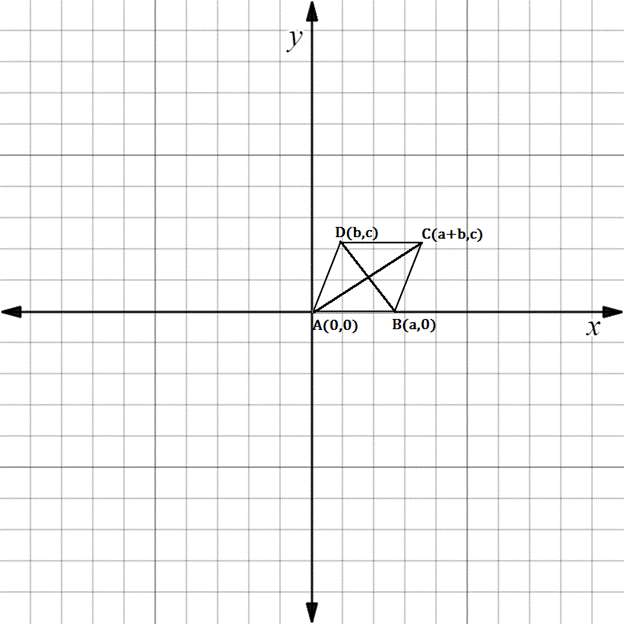Chapter 10.3, Problem 31EElementary Geometry For College St...

7th Edition
Alexander + 2 others
ISBN: 9781337614085

Solutions

Chapter
SectionElementary Geometry For College St...

7th Edition
Alexander + 2 others
ISBN: 9781337614085
Textbook Problem

Which formula would you use to establish each of the following claims?a) A C - ⟘ D B - b) A C - = D B - c) D B - and A C - bisect each otherd) A C - ║ D B -A B C D is parallelogram.

(a)

To determine

To find:

The formula used to establish AC-DB-.

Explanation

The given condition is AC-DB-.

If AC- is perpendicular to DB<

(b)

To determine

To find:

The formula used to establish AC-=DB-.

(c)

To determine

To find:

The formula used to establish DB- and AC- bisect each other.

(d)

To determine

To find:

The formula used to establish AC-DB-.

Still sussing out bartleby?

Check out a sample textbook solution.

See a sample solution

The Solution to Your Study Problems

Bartleby provides explanations to thousands of textbook problems written by our experts, many with advanced degrees!

Get Started